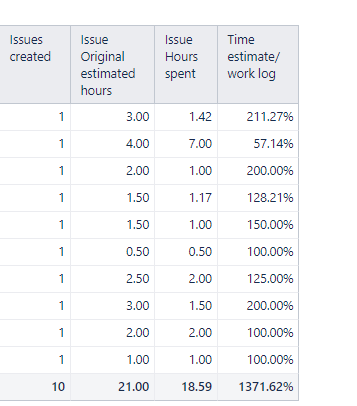# How to customize the total option

Hi everyone I am new to eazybi I am struggling with totals of some values below is the report where I have two measures issues with original estimate and issue hours spend I have 10 issues when I divide the original estimate with hours spend instead of divided the total values eazybi divide each issue and the total it which gives my result wrong I just want to divide the total values kindly someone help me with thisHi @Umar

It seems that you reached out to us directly and we sent a reply to you directly from our support email.
I will post the solution here as well, in case other eazyBI users have ran into a same scenario and are looking for solutions.
Here is a formula I shared with you:

``````CASE WHEN [Issue].CurrentHierarchyMember.Name = '\$total_aggregate'
THEN
Sum(
VisibleRowsSet()
,
[Measures].[Issue Original estimated hours]
)/
Sum(
VisibleRowsSet()
,
[Measures].[Issue Hours spent]
)
ELSE
[Measures].[Issue Original estimated hours]/[Measures].[Issue Hours spent]
END
``````

Best wishes,

Elita from support@eazybi.com